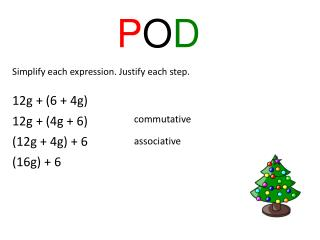DownloadDownload PresentationP O D

# P O D

Download Presentation## P O D

- - - - - - - - - - - - - - - - - - - - - - - - - - - E N D - - - - - - - - - - - - - - - - - - - - - - - - - - -
##### Presentation Transcript

1. POD Simplify each expression. Justify each step. 12g + (6 + 4g) 12g + (4g + 6) commutative (12g + 4g) + 6 associative (16g) + 6

2. POD Use mental math. 5 × 123 5(100) + 5(20) + 5(3) 500 + 100 + 15 615

3. The Distributive Property states that to multiply a sum or difference by a number, multiply each term inside the parenthesis by the number outside the parenthesis. a( b + c ) = ab + ac a( b – c ) = ab − ac

4. 2 ( y + 2) = 2(y) +2(2) = 2y + 4 The expressions 2(y+2) and 2y+4 are equivalent expressions. No matter what y is, these expressions have the same value.

5. 8(-9+4) = 8(-9) + 8(4) = -72 + 32 = -40

6. Whiteboard time 5(-9 + 11) 5(-9) + 5(11) -45 + 55 10

7. Whiteboard time 7(10 − 5) 7(10) − 7(5) 70 − 35 35

8. Whiteboard time (12 − 8)9 9(12) − 9(8) 108 − 72 36

9. Whiteboard time 4(x + 7) 4(x) +4(7) 4x + 28

10. Whiteboard time 5(-3x + 7y) 5(-3x) + 5(7y) -15x + 35y

11. Whiteboard time Use mental math to solve. 6 × 15 6(10) + 6(5) 60+ 30 90

12. Whiteboard time Use mental math to solve. 5 × 35 5(30) + 5(5) 150+ 25 175

13. Whiteboard time Use mental math to solve. 3 × 124 3(100) + 3(20) + 3(4) 300 + 60 + 12 372

14. Whiteboard time -5( -2 – 18) -5(-2) − (-5)(18) 10 − (-90) 100

15. Whiteboard time 3(-4 + 12) 3(-4) + 3(12) -12 + 36 24

16. Whiteboard time 12( 2x + 3) 12(2x) + 12(3) 24x +36

17. Whiteboard time -2g( 4y + 2) -2g(4y) + -2g(2) -8gy + -4g

18. Whiteboard time Use mental math to solve. 7 × 14 7(10) + 7(4) 70+28 98

19. Whiteboard time Use mental math to solve. 9 × 22 9(20) + 9(2) 180+ 18 198

20. Mr. Ito needs to buy batting helmets for the baseball team. The helmets he plans to buy are \$19.95 each. Find the total cost if Mr. Ito needs to buy 9 batting helmets for the team. Rename \$19.95 as \$20.00−\$0.05 9(20 − 0.05) 9(20) − 9(0.05) \$180 − \$0.45 \$179.55